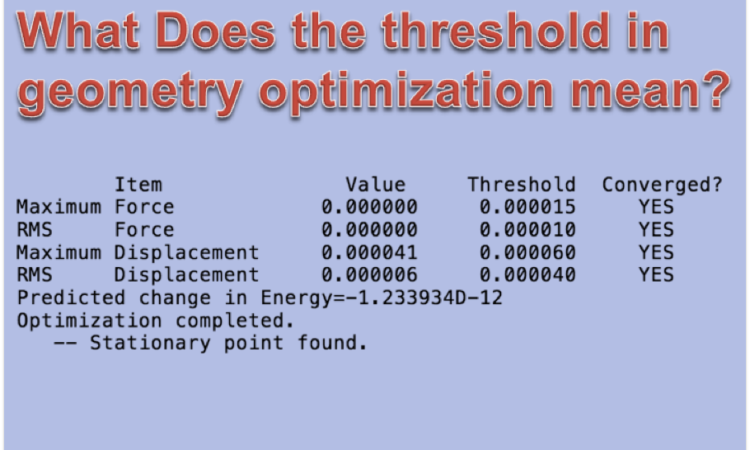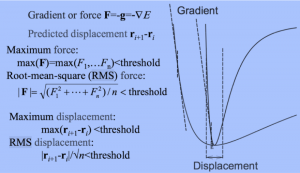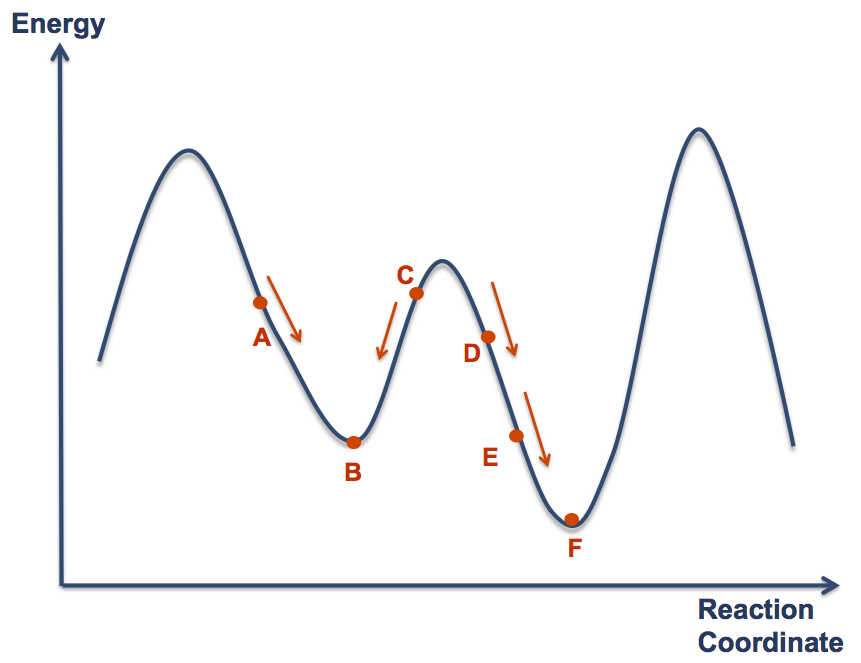# What Does the threshold in geometry optimization mean?

Nowadays, not only computational chemists but also experimental scientists can do calculations. However, most of them don’t know how to read log file…

For example, optimization.

Four values displayed in log file during structure optimization in Gaussian. When all of them become lower than the threshold, the structure optimization is completed.

So what does each of the four values mean?

Also, how can you perform structure optimization more efficiently?

Gaussian performs structure optimization using Berny algorithm. In other words, the gradient is obtained by first derivative of the potential energy phase (PES), and it will search for the flat point where the gradient is nearly 0. On the other hand, the disadvantage of Berny algorithm is that as you move closer to the convergence point, the step width decreases and it slows down. In other words, the closer you get to the bottom of the valley, the slower it will be.

Based on the above, when mathematically considering structure optimization, we can write the conceptual diagram as follows.The first derivative on PES is Force. This value represents the gradient of PES.

Force: F = – ∇E

PES has 3N-6 dimensions, so there are 3N-6 Fs. In the above figure, the equation: max (F) <threshold, representing the Maximum force, shows that the gradient of PES is close to 0 in all vibration mode. This means molecule is at the state of local minima or local maxima.

RMS stands for Root Mean Square. If the size of RMS is less than or equal to the threshold value, it means average value of 3N-6 of Fs are less than the threshold value. When optimizing the structure, the “RMS Force” meet the threashold requirement, displaying “YES”, faster than the “Maximum Force”. It should be displayed as YES.

Displacement is an indicator of how much coordinates move in the search on PES. When moving from one point “r” on the PES to the next point “r + 1” during the structural optimization calculation, the coordinates can be represented by a function of eigenvalues, eigenvectors and F. When the difference between “r + 1” and “r” obtained by this calculation falls below the threshold, it can be said that it is at local minima or local maxima on PES. This is consistent with the feature of the Berny method that the step width decreases as approaching the valley bottom.

A molecule in which this displacement is hard to be converged can be a case where the mountains or valleys on PES are super steep or very shallow. In other words, convergence point comes between a certain point “r” and the point “r + 1” after the movement, which means that it is difficult to converge. In such a case, reducing the step width of the structure optimization sometimes work.

Also, convergence is difficult even when the structure is super flexible. In such a case, lowering the level of the basis function may make the gradient of PES moderate and solve the problem.

#### Structural optimization tips

As described above, in the structure optimization, the structure is gradually changed based on the gradient information of the PES. Therefore, the molecule will be changed towards the nearest stable structure (local minima) from the initial coordinates of the calculation. The figure is shown below.In the above figure, the most stable point (global minima) is F, but if you specify A or C as the initial structure, B will be obtained as the optimization result. The program can not judge where the global minima is, because it judges only by gradient and moves in the direction where energy becomes smaller.

If you want to obtain F, you need to specify D or E as the initial structure. In this case of using D as an initial structure, the calculation ends earlier than the case where E is an initial structure. As the Quantum Mechanics calculation requires expensive calculation cost, it is better to make the initial structure as close as possible to the product.

So, how can we get the initial structure?

One way is to use a cheap calculation method.

Since it is very time consuming to make a structure like E or F by hand, first write the structure of D and bring it closer to E by semi-empirical method (PM 6 etc.). Furthermore, if you optimize with B3LYP / 6-31G (d) etc. and structure optimization with B3LYP / 6-31 + G (d, p) etc. based on the result, you can proceed your calculations much more efficiently .

In Quantum Mechanics calculation, the initial structure is very important, and it affects the calculation cost and the result!

#### How can we judge the result ?

Knowledge of organic chemistry is necessary to judge whether the obtained structure is really a global minima or not. If you read like this, you may think that subjectivity would come in as much as you thought of computational chemistry, but that is right!

Therefore, when you perform optimization, you need to use combined methods, such as conformational search. Nowadays, many computational chemists use MM calculation before the optimization. MM calculation provides you a thousands of comformers, so you also need to set the threashold for it.

If you prefer QM conformational search, I recommend you to use GRRM’s QM conformational search.

#### Related Artcles

This site uses Akismet to reduce spam. Learn how your comment data is processed.